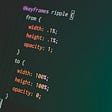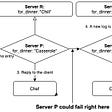# Subsets — I

Given a set of distinct integers, nums, return all possible subsets (the power set).

Note: The solution set must not contain duplicate subsets.

Example:

`Input: nums = [1,2,3]Output:[  ,  ,  ,  [1,2,3],  [1,3],  [2,3],  [1,2],  []]`

# THREE DIFFERENT SOLUTIONS

For each element, I have two choices whether to keep it or not, I execute my choice and then ask recursion to do the remaining work.

Instead of saying that the decision(recursive) tree will help to understand what I just said, I would say, I reached this solution by drawing the recursive tree. It is highly recommended to solve recursion/backtracking by drawing trees. Once you draw the right decision tree, the logic and code are not too far.

`Take example of [1,2,3].([ans],[nums])                          ([],[1,2,3])         (choose 1)  /                  \ (don't choose 1)                    /                    \              (,[2,3])                ([],[2,3])      (choose 2)/ \(don't choose 2)  (choose 2)/ \(don't choose 2)                   /   \                         /   \       ([1,2],)  (,)          (,)   ([],)(choose 3)/ \(don't choose 3) ....................................         /   \  ([1,2,3],[])  ([1,2],[]) ([1,3],[]) ([1,3],[]).......(Base cases when nums is empty)PS: Please try this on paper and pardon me if the tree doesn't look good :)`

FINALLY THE CODE :

`//BACKTRACKING 1class Solution {public:    vector<vector<int>> ans;    void subsets(vector<int>& nums, vector<int> &currset, int start){               //base case        if(start == nums.size()){            ans.push_back(currset);            return;        }                        //for every elt in nums, we have 2 choices                 //choice 1 : keep it in currans        currset.push_back(nums[start]);        subsets(nums,currset,start+1);                //choice 2 : Don't keep it in currans        currset.pop_back();        subsets(nums,currset,start+1);                }        vector<vector<int>> subsets(vector<int>& nums) {        vector<int> currans = {};                subsets(nums,currans,0);                return ans;    }};`

For each element in nums, I will push back the current number into my current set (`currset`) and then ask recursion to work on the sets starting from this current number and then backtrack by popping back the current number. For example, let’s say for nums =[1,2,3], I push back into my currset the 0th number i.e, 1 and ask recursion to work on numbers from 1st index(keep in mind, we already pushed 1 into our currset, so this recursive call is gonna work on {1} as currset and will ultimately return by pushing in all the subsets starting from 1, that are {,[1,2],[1,2,3],[1,3]}) and when this work is done, backtrack and pop_back the currset and work on the numbers starting from index 1 (i.e, 2 ) resulting in adding all the subsets starting from 2, and then backtrack and again work on the numbers starting from index 2(i.e, 3) resulting in adding all the subsets starting from 3, and hence the for loop.

`// BACKTRACKING 2class Solution {public:    vector<vector<int>> ans;        void subsets(vector<int> nums, int start, vector<int> currset){         ans.push_back(currset)        //base case        if(start == nums.size()){            return;        }          for(int j = start; j < nums.size(); j++){            currset.push_back(nums[j]);            subsets(nums,j+1,currset);            currset.pop_back();        }    }    vector<vector<int>> subsets(vector<int>& nums) {        subsets(nums,0,{});        return ans;    }};`

Using `[1, 2, 3]` as an example, the iterative process is like:

1. Initially, one empty subset `[[]]`
2. Adding `1` to `[]`: `[[], ]`;
3. Adding `2` to `[]` and ``: `[[], , , [1, 2]]`;
4. Adding `3` to `[]`, ``, `` and `[1, 2]`: `[[], , , [1, 2], , [1, 3], [2, 3], [1, 2, 3]]`.
`//ITERATIVEclass Solution {public:    vector<vector<int>> subsets(vector<int>& nums) {        vector<vector<int>> ans= {{}};        for (int num : nums) {            int n = ans.size();            for (int i = 0; i < n; i++) {                ans.push_back(subs[i]);                 ans.back().push_back(num);            }        }        return ans;    }};`

# Subsets — II

Given a collection of integers that might contain duplicates, nums, return all possible subsets (the power set).

Note: The solution set must not contain duplicate subsets.

Example:

`Input: [1,2,2]Output:[  ,  ,  [1,2,2],  [2,2],  [1,2],  []]`

Think about the 2nd backtracking solution, we talked about for subsets — I, the solution for this problem is also hidden in the recursive tree of that solution. Ok, so if we talk about distinct elements as earlier, (let’s say nums = [1,2,3]), if you remember from the above solution, we pushed 1 in currset and asked for all the sets starting with 1 and then backtracked, which gives us {,[1,2],[1,2,3],[1,3]} and then we proceeded to subsets starting with 2.
Now, if we consider the case of duplicate elements, (let’s say nums = [1,1,2]), if we have made the recursive call for numbers starting with 1(0th index), we do not need to make another call for numbers starting with 1(1st index), so we, `continue` if we find such a situation.

PS: nums are sorted beforehand to make sure that our condition `nums[i] == nums[i-1]` is able to catch and skip the duplicate recursive calls.

`class Solution{public:   vector<vector<int>> ans;  void helper(vector<int> &nums, vector<int> &currans, int start)  {    ans.push_back(currans);for (int i = start; i < nums.size(); i++)    {      if (i > start && nums[i] == nums[i - 1])        continue;        currans.push_back(nums[i]);        helper(nums,currans,i+1);        currans.pop_back();    }  }vector<vector<int>> subsetsWithDup(vector<int> &nums)  {    sort(nums.begin(), nums.end());    vector<int> currans = {};    helper(nums, currans, 0);    return ans;  }};`

# SEE YOU IN THE NEXT PART WITH QUESTIONS RELATED TO PERMUTATIONS :)

--

--

--

Software Developer working to become a better Software Developer everyday :)

Love podcasts or audiobooks? Learn on the go with our new app.

## Bitrise Android Environment Variables## How does a Computer store data?## Data Structures with Go## Dart Programming — Tips and Tricks## Learning To Code Changed Me## PIRO: An improved synchronization model for strongly-consistent primary-replica systems## Manvi Tyagi

Software Developer working to become a better Software Developer everyday :)

## Let the cyber punk era commence## Never succeed in startups: An Ultimate Guide.## Who is Luke Snow? An interview with the Physical and Digital Art genius